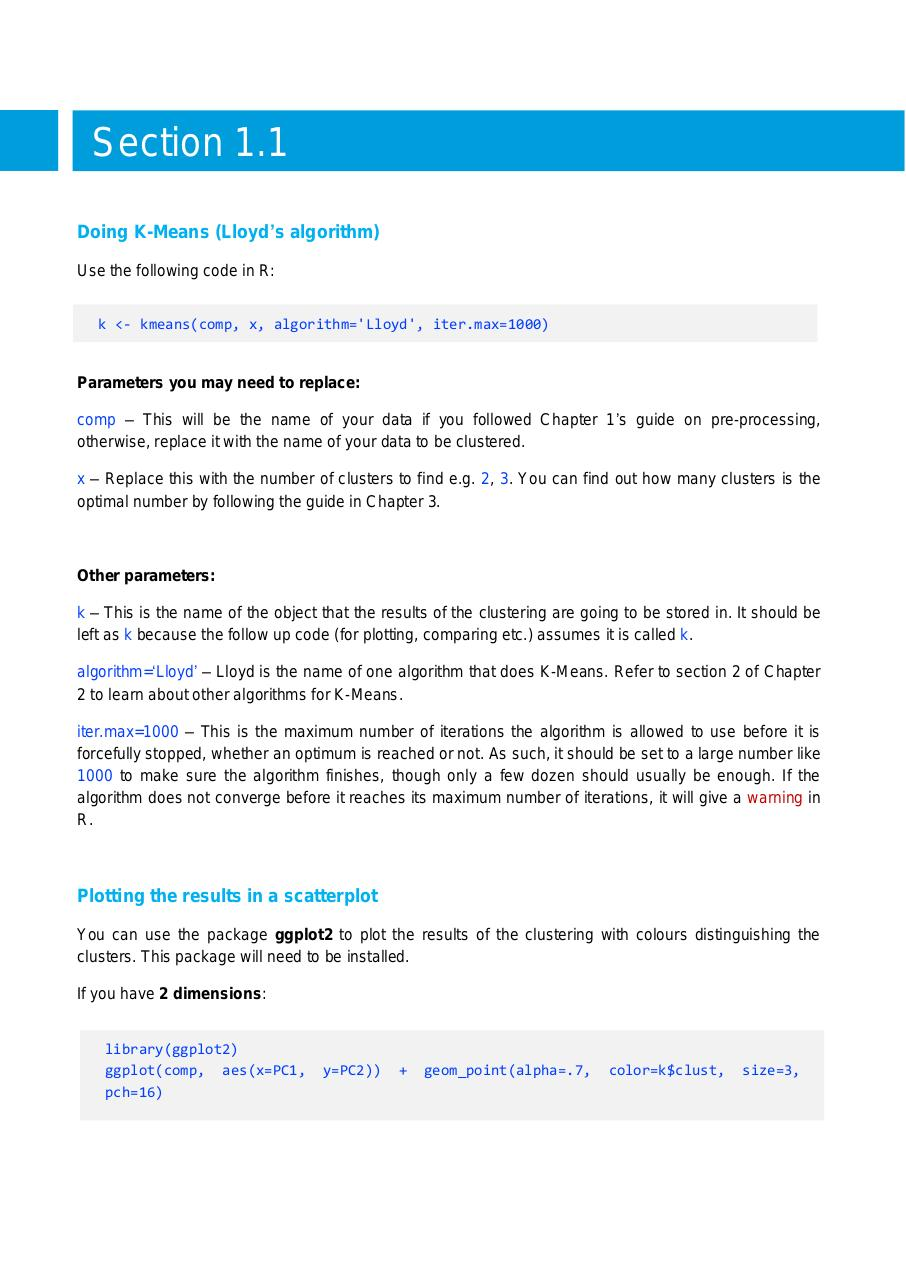PDF Archive

Easily share your PDF documents with your contacts, on the Web and Social Networks.

2B R Code.pdfPage 1 2 345102

Text preview

Section 1.1
Doing K-Means (Lloyd’s algorithm)
Use the following code in R:
k &lt;- kmeans(comp, x, algorithm='Lloyd', iter.max=1000)

Parameters you may need to replace:
comp – This will be the name of your data if you followed Chapter 1’s guide on pre-processing,
otherwise, replace it with the name of your data to be clustered.
x – Replace this with the number of clusters to find e.g. 2, 3. You can find out how many clusters is the
optimal number by following the guide in Chapter 3.

Other parameters:
k – This is the name of the object that the results of the clustering are going to be stored in. It should be
left as k because the follow up code (for plotting, comparing etc.) assumes it is called k.
algorithm=‘Lloyd’ – Lloyd is the name of one algorithm that does K-Means. Refer to section 2 of Chapter
2 to learn about other algorithms for K-Means.
iter.max=1000 – This is the maximum number of iterations the algorithm is allowed to use before it is
forcefully stopped, whether an optimum is reached or not. As such, it should be set to a large number like
1000 to make sure the algorithm finishes, though only a few dozen should usually be enough. If the
algorithm does not converge before it reaches its maximum number of iterations, it will give a warning in
R.

Plotting the results in a scatterplot
You can use the package ggplot2 to plot the results of the clustering with colours distinguishing the
clusters. This package will need to be installed.
If you have 2 dimensions:

library(ggplot2)
ggplot(comp, aes(x=PC1,
pch=16)

y=PC2))

+

geom_point(alpha=.7,

color=k\$clust,

size=3,